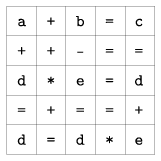Mathematical and Physical Journal
for High Schools
Issued by the MATFUND Foundation
 Already signed up? New to KöMaL?

#Problem S. 31. (December 2007)

S. 31. Someone made up a 5×5 table containing equations. Each cell of the table contains either a basic arithmetic operation (any of the 4 operations), a variable (a single letter) or the equality sign.

See the example:When reading rows (from left to right), columns and the two diagonals (downwards) of the table, we get a system of 8 equations. During the solution of this system, one can always find an equation from which a suitable unknown can be expressed (independently of the other equations), or a suitable simple relation between two unknowns can be derived.

In the example above, one sees from the last line that d=0 or e=1. The first column implies a=0, so b=c due to the first row. Proceeding in a similar way, the solution is a=d=0 with b=c=e=arbitrary; or a=0, e=1 and b=c=1+d with d arbitrary.

Write your program to solve an arbitrary (syntactically correct) table. The first input parameter of your program is the name of the text file containing the table. The input file consists of 5 rows. Each row contains the successive entries of a row in the table (that is, mathematical symbols or unknowns) separated by a space. The output of your program on the standard output should consist of a list of values of the variables in alphabetical order (e.g. a=3.25'' or the value of a is arbitrary''). If the system can not be solved, a warning should be issued.

The source code of your program (s31.pas, s31.cpp, ...) together with a short documentation (s31.txt, s31.pdf, ...) should be submitted, also containing a short description of your code and the name of the developer environment to use.

Proposed by András Tálas, 12th grade student, Budapest

(10 pont)

Deadline expired on May 15, 2008.

### Statistics:

 2 students sent a solution. 4 points: 2 students.

Problems in Information Technology of KöMaL, December 2007